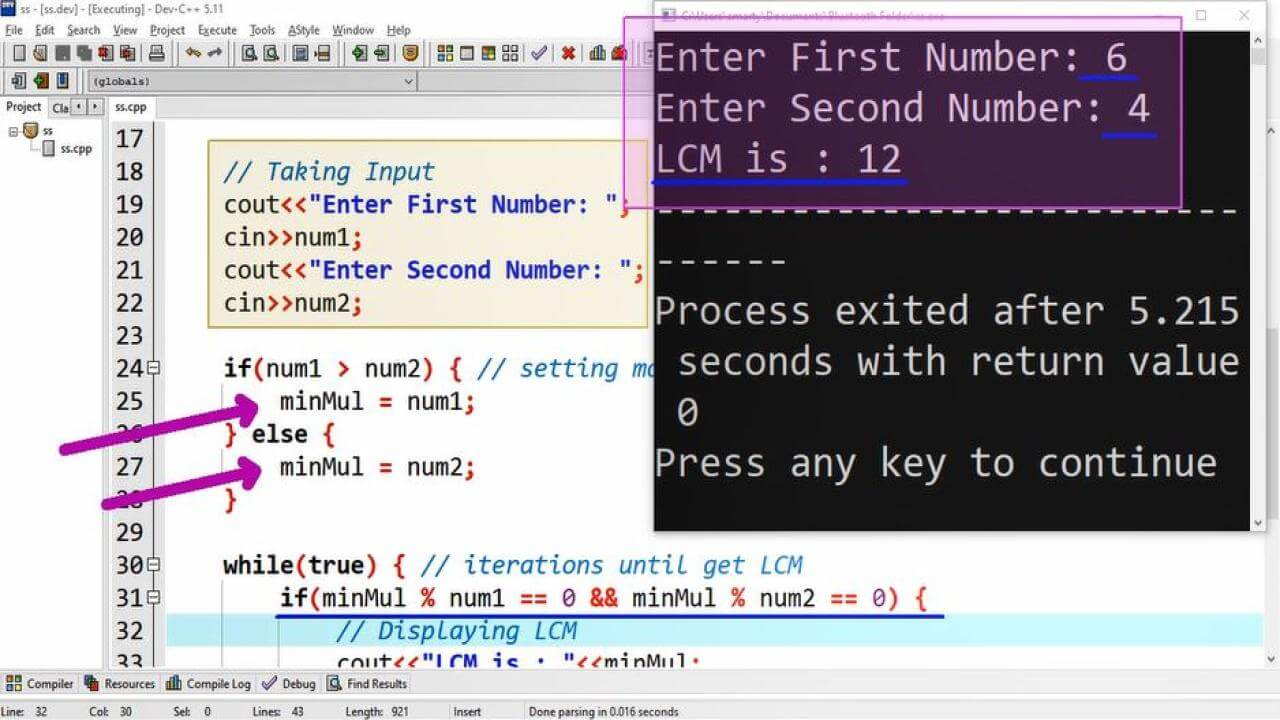# Lcm Program Example using CPP

## Source Code:

#include <iostream>
using namespace std;

/*
Least Common Multipliers
For Example:
Multipliers of given numbers
3 = 3,6,9,12,15,18,21,.......
4 = 4,8,12,16,20,24,28,......
*/

int main(int argc, char** argv) {
//Variable Declarations
int num1;  // To get First Input
int num2;  // To get Second Input
int minMul; // For repated factors

// Taking Input
cout<<"Enter First Number: ";
cin>>num1;
cout<<"Enter Second Number: ";
cin>>num2;

if(num1 > num2) { // setting maximum as dividant
minMul = num1;
} else {
minMul = num2;
}

while(true) { // iterations until get LCM
if(minMul % num1 == 0 && minMul % num2 == 0) {
// Displaying LCM
cout<<"LCM is : "<<minMul;
break;  // break is used to terminate loop
}
// incrementing minimum multiplier by 1
minMul = minMul + 1;
}

return 0;
}

##Working:

This program example is compiled and tested using Dev-C++ code editor. LCM (Least Common Multiple) is the smallest factor among the input numbers. LCM can be calculated using multiple methods such as.

1. Prime Factorization Method
2. List of Multiples Method
3. Division Method

Here in this source code we use list of multiples method to find the LCM of given numbers. We can calculate LCM of multiple numbers may be stored in an array. For understanding here we only take input in two variables of int data type. The list of variable along with their description is provided below.

 Sr. Identifier Data Type Description 1 num1 int num1 is used to take first number input. 2 num2 int num2 is used to take second number input. 3 minMul int variable used to get maximum of two numbers.

Here the minMul variable is used to store the maximum of input variable. After storing maximum input we set an infinte loop that will check eithe the minMul variable value is completely divisible by both input variables (num1 nd num2). If this condition true then we terminate this loop using break statement.